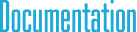#SpatialMatrix6f Class Reference

## Detailed Description

6D spatial matrix with float type elementsInheritance diagram for SpatialMatrix6f:

## Public Member Functions

Initialization methods
void Set (const Matrix3< float > &_R, const Point3< float > &_r)

void Set (const Matrix3< float > &m11, const Matrix3< float > &m21, const Matrix3< float > &m12, const Matrix3< float > &m22)

void Set (const SpatialTrans6< float > &tm)

void SetTensorProduct (const SpatialVector6< float > &p1, const SpatialVector6< float > &p2)

void SetIdentity ()

void Zero ()

Unary operators
SpatialMatrix6 operator- () const

SpatialMatrix6 operator- (const SpatialMatrix6 &mat) const

SpatialVector6< float > operator* (const SpatialVector6< float > &p) const

SpatialMatrix6 operator* (const SpatialMatrix6 &mat) const

SpatialMatrix6 operator* (float t) const

SpatialMatrix6 operator+ (const SpatialMatrix6 &mat) const

SpatialMatrix6 operator/ (float t) const

## Public Attributes

Matrix3< float > m 

## Assignment operators

void operator*= (const SpatialMatrix6 &mat)

void operator*= (float t)

void operator+= (const SpatialMatrix6 &mat)

void operator-= (const SpatialMatrix6 &mat)

void SetMatrix (Matrix3< float > &m, const Point3< float > &p1, const Point3< float > &p2)

## § Set() [1/3]

 void Set ( const Matrix3< float > & _R, const Point3< float > & _r )
inherited

Sets the matrix as the outer product of two vectors.

## § Set() [2/3]

 void Set ( const Matrix3< float > & m11, const Matrix3< float > & m21, const Matrix3< float > & m12, const Matrix3< float > & m22 )
inherited

Sets the matrix as the outer product of two vectors.

## § Set() [3/3]

 void Set ( const SpatialTrans6< float > & tm )
inherited

Sets the matrix as the outer product of two vectors.

## § SetTensorProduct()

 void SetTensorProduct ( const SpatialVector6< float > & p1, const SpatialVector6< float > & p2 )
inherited

Sets the matrix as the outer product of two vectors.

## § SetIdentity()

 void SetIdentity ( )
inherited

Sets the matrix as the outer product of two vectors.

## § Zero()

 void Zero ( )
inherited

Sets the matrix as the outer product of two vectors.

## § m

 Matrix3 m
inherited

Matrix data in column major order.